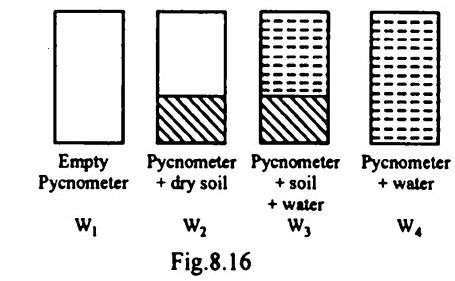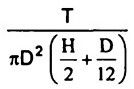top of pageSearch

# Soil Mechanics (301-320)

Updated: Apr 27, 2020

301. An undrained triaxial compression test is carried out on a saturated clay sample under a cell pressure of 100 kN/m². The sample failed at a deviator stress of 200 kN/m². The cohesion of the given sample of clay is 100 kN/m²

302. Which one of the following planes is most likely to be the failure plane in sandy soils? Plane, with the maximum angle of obliquity

303. A retaining wall retains a c-§ soil backfill. Tension cracks have occurred in

the backfill. The total active earth pressure is given by (y = unit weight of soil, H =

height of retaining wall, kA = coefficient of active earth pressure, c' = cohesion of

soil)304. In which one of the following pairs of soil types would one anticipate negative pore pressure, when subjected to shearing ?

dense sand and overconsolidated clay

305. In a saturated soil deposit having a density of 22 kN/m³, the effective normal

stress on a horizontal plane at 5 m depth 60 kn/m²

306. A fully saturated clay specimen is placed in a consolidometer and subjected to a loading of 200 kN/m². After a period of time it was found that the average pore pressure in the specimen was 70 kN/m². The percentage of consolidation reached by then was 65

307.The virgin compression curve for a particular soil is shown in Fig.8.15. The

compression index of the soil is

0.50

308. A 2 m wide strip footing rests at a depth of 2 m below ground surface in a clay deposit where water table is at ground surface, c = 3 t/m² and yₛₐₜ =2 t/m³ The

best estimate of the net ultimate load which the footing can carry is 18 t/m

309. In Newmark's influence chart for stress distribution, there are ten concentric

circles and ten radial lines. The influence factor of the chart is 0.01

310.

Friction pile- Stiff clay Batter pile- Lateral load Tension pile- Uplift load Compaction pile- Loose granular soil

311.As per Indian code practice, the frequency ratio (ratio of operating frequency of a machine to the natural frequency of soil) should not be within the range of 0.5 to 1.5

312. Figures 8.16 indicate the weights of different pycnometersThe specific gravity of solids is given by313.A soil sample has a shrinkage limit of 10% and specific gravity of soil solids as

2.7. The porosity of the soil at shrinkage limit is 21.2%

314. A soil has a discharge velocity of 6 x 10⁻⁷ m/s and a void ratio of 0.5. Its seepage

velocity is 18 x 10⁻⁷ m/s

315. If during a permeability test on a soil sample with a falling head permeameter,

equal time intervals are noted for drop of head from h to h and again from hto h then which one of the following relations would hold good?. h₂² = hh

316.For conducting a Standard Proctor compaction test, the weight of hammer (P

in kg), the fall of hammer (Q in mm), the number of blows per layer (R) and the

number of layers (S) required are respectively P-2.6,Q-310,R-25,S-3

317. A flownet is drawn to obtain exit gradient, uplift pressure and seepage quantity

318.A laboratory vane shear test apparatus is used to determine the shear strength of a clay sample and only one end of the vane takes part in shearing the soil. If T = applied torque, H = height of vane and D = diameter of the vane, then the shear strength of the clay is given by319. A retaining wall retains a sand strata with Φ = 30° upto its top. If a uniform surcharge of 12 t/m is subsequently put on the sand strata, then the increase in the lateral earth pressure intensity on the retaining wall will be 4t/m²

320. Consider the following assumptions for slope stability analysis.

1. Friction is fully mobilized.

2. Total stress analysis is used.

3. Resultant 4R' is tangential to the friction circle